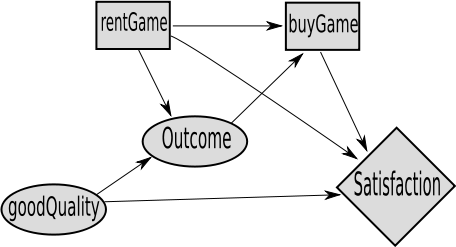Practice Exercise 9.B
Sequential Decisions

## 2: Learning Goals

• Represent sequential decision problems as decision networks and explain the no-forgetting property.
• Verify whether a possible world satisfies a policy and define the expected value of a policy.
• Compute the number of policies for a decision problem.
• Compute the optimal policy by Variable Elimination.

## 3: Directed Questions

1. How is a sequential decision problem different from a one-off decision problem? [solution]

2. What types of variables are contained in a decision network? [solution]

3. What can arcs represent in a decision network? Relate this to the types of variables in the previous question. [solution]

4. What is a no-forgetting decision network? [solution]

5. Define decision function and policy. [solution]

6. A possible world specifies a value for every random variable and decision variable. Given a policy and a possible world, how do we know if the possible world satisfies the policy? [solution]

7. To find an optimal policy, do we need to enumerate all of the policies? Why or why not? [solution]

## 4: Exercise: Wii Games

Miranda is an enthusiastic gamer, spending quite a bit of time playing Wii video games and a fair amount of money buying them. She notices that her neighbourhood video store rents Wii games for much less than the cost of buying one. She realizes that renting the games might be a good way to test them out before she decides whether or not to buy them. The following figure represents her decision problem:Based on prior experience, Miranda expects that about 80% of video games will be good quality and the other 20% she won't care for. Based on her previous experiences renting video games, she also knows the following information:

P (Outcome = likesGame|goodQuality = True) = 0.85
P (Outcome = likesGame|goodQuality = False) = 0.10

The rental period is so short that it's not always possible to get a reliable estimate of whether the game is of good quality.

Below are the utilities for various outcomes of the decision process. You can think of the utilities as representing a combination of gaming enjoyment and money saved (Satisfaction).

T T T 80.0
T T F -100.0
T F T 30.0
T F F -30.0
F T T 100.0
F T F -80.0
F F T 0.0
F F F 0.0
1. If we carry out the variable elimination algorithm, what are the initial factors? [solution]

2. Which decision variable is eliminated first, and why? [solution]

3. How is that decision eliminated? [solution]

4. After that decision is eliminated, which variable is eliminated next, and why? [solution]

5. What is the optimal policy for this decision problem? [solution]

6. What is the expected utility of following the optimal policy? [solution]
Use the belief and decision networks tool to represent and solve this decision problem, and to check your answers. The representation we have used is in the following file: http://www.aispace.org/exercises/wii.xml.

## 5: Learning Goals Revisited

• Represent sequential decision problems as decision networks and explain the no-forgetting property.
• Verify whether a possible world satisfies a policy and define the expected value of a policy.
• Compute the number of policies for a decision problem.
• Compute the optimal policy by Variable Elimination.